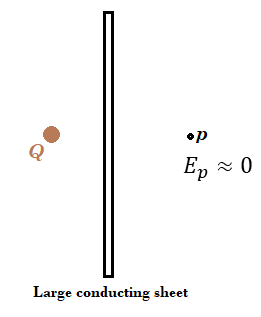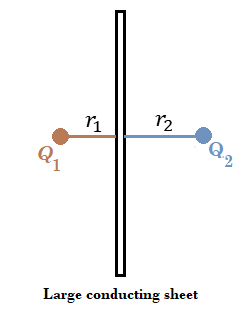# Electric Field Shielding by Conducting Sheets

• I
• NikhilRG
In summary, a thin conducting sheet can significantly shield an electric field caused by a point charge. If the sheet is large enough, then Gauss's law holds true which means that the shielding is greatly enhanced.f

#### NikhilRG

TL;DR Summary
Thin conducting sheet placed in front of a point charge.
Dear Experts,
When a thin conducting sheet with no charge on is placed at a certain distance from a point charge, does it shield the electric field caused due to the point charge from reaching the other side of the sheet. As an extension of that idea, when a conducting sheet or slab is placed in the space between two point charges in space, how will the interaction between the point charges be affected by the introduction of the conducting sheet between them.

Hello @NikhilRG ,##\qquad##!

When a thin conducting sheet with no charge on is placed at a certain distance from a point charge, does it shield the electric field caused due to the point charge from reaching the other side of the sheet.
No it does not. A charge distribution of opposite charge will build up on the side towards the charge and that leaves the other side with a charge of the same sign.

A comparable situation is a charge within a conducting shell.

As an extension of that idea, when a conducting sheet or slab is placed in the space between two point charges in space, how will the interaction between the point charges be affected by the introduction of the conducting sheet between them.
The eletric field will be influenced because the sheet is an equipotential surface.

##\ ##

•vanhees71 and NikhilRG
When a thin conducting sheet with no charge on is placed at a certain distance from a point charge, does it shield the electric field caused due to the point charge from reaching the other side of the sheet.
I believe there can be significant shielding for a large sheet. The larger the sheet, the better the shielding.The net electric field at point ##p## will be weak if ##Q## and ##p## are near the large sheet and away from the sheet edge. If the sheet is infinite, then the field will be zero at all points to the right of the sheet.

As an extension of that idea, when a conducting sheet or slab is placed in the space between two point charges in space, how will the interaction between the point charges be affected by the introduction of the conducting sheet between them.
If the sheet is small, then it would be complicated to calculate the forces.

For a large sheet where the charges are near the sheet and away from the edge of the sheet, each charge will feel a net force toward the sheet independent of the signs of the charges.For an infinite sheet I think we would have

$$F_1 = \frac 1 {4 \pi \epsilon_0} \frac{Q_1^2}{ (2r_1)^2} \qquad \mathrm{and} \qquad F_2 =\frac 1 {4 \pi \epsilon_0} \frac{Q_2^2}{(2r_2)^2}$$
Here, the force felt by a charge can be thought of as the attraction of the charge to its image charge. ##F_1## is independent of ##Q_2## and ##F_2## is independent of ##Q_1##.

Posts #2 and #3 are not conflicting: a conducting sheet definitely influences the electric field. Depending on relative size of sheet and distance between sheet and charge, there is a region where there is an amount of shielding. But the Gauss theorem (or Gauss's law) holds true in all cases.

(Which also means that the shielding is enhanced considerably by grounding the sheet!)

##\ ##

Last edited:
•SammyS